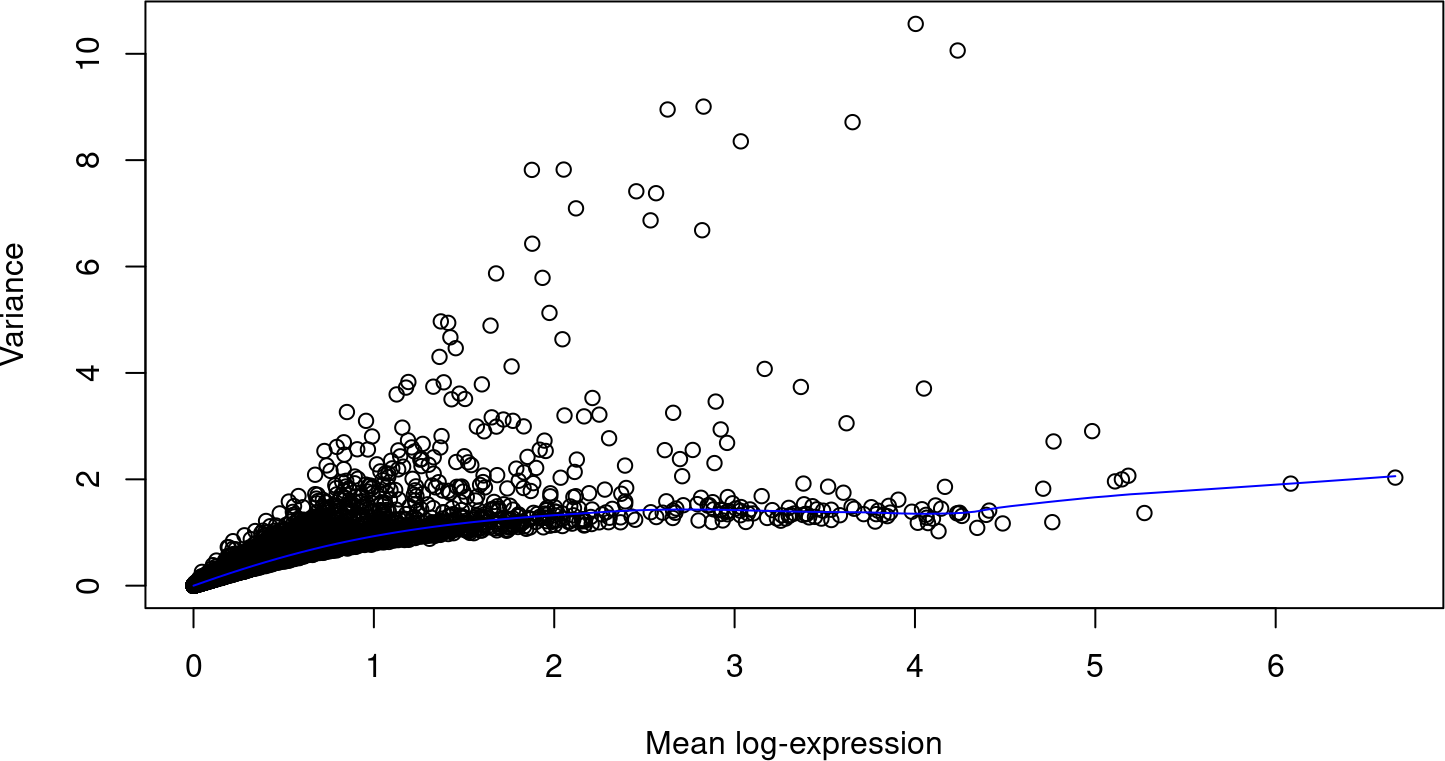# 1 Introduction

Single-cell RNA sequencing (scRNA-seq) is a widely used technique for profiling gene expression in individual cells. This allows molecular biology to be studied at a resolution that cannot be matched by bulk sequencing of cell populations. The scran package implements methods to perform low-level processing of scRNA-seq data, including cell cycle phase assignment, variance modelling and testing for marker genes and gene-gene correlations. This vignette provides brief descriptions of these methods and some toy examples to demonstrate their use.

Note: A more comprehensive description of the use of scran (along with other packages) in a scRNA-seq analysis workflow is available at https://osca.bioconductor.org.

# 2 Setting up the data

We start off with a count matrix where each row is a gene and each column is a cell. These can be obtained by mapping read sequences to a reference genome, and then counting the number of reads mapped to the exons of each gene. (See, for example, the Rsubread package to do both of these tasks.) Alternatively, pseudo-alignment methods can be used to quantify the abundance of each transcript in each cell. For simplicity, we will pull out an existing dataset from the scRNAseq package.

``````library(scRNAseq)
sce <- GrunPancreasData()
sce``````
``````## class: SingleCellExperiment
## dim: 20064 1728
## metadata(0):
## assays(1): counts
## rownames(20064): A1BG-AS1__chr19 A1BG__chr19 ... ZZEF1__chr17
##   ZZZ3__chr1
## rowData names(2): symbol chr
## colnames(1728): D2ex_1 D2ex_2 ... D17TGFB_95 D17TGFB_96
## colData names(2): donor sample
## reducedDimNames(0):
## mainExpName: endogenous
## altExpNames(1): ERCC``````

This particular dataset is taken from a study of the human pancreas with the CEL-seq protocol (Grun et al. 2016). It is provided as a `SingleCellExperiment` object (from the SingleCellExperiment package), which contains the raw data and various annotations. We perform some cursory quality control to remove cells with low total counts or high spike-in percentages:

``````library(scuttle)
qcstats <- perCellQCMetrics(sce)
qcfilter <- quickPerCellQC(qcstats, percent_subsets="altexps_ERCC_percent")
sce <- sce[,!qcfilter\$discard]
summary(qcfilter\$discard)``````
``````##    Mode   FALSE    TRUE
## logical    1291     437``````

Cell-specific biases are normalized using the `computeSumFactors` method, which implements the deconvolution strategy for scaling normalization (Lun, Bach, and Marioni 2016).

``````library(scran)
clusters <- quickCluster(sce)
sce <- computeSumFactors(sce, clusters=clusters)
summary(sizeFactors(sce))``````
``````##     Min.  1st Qu.   Median     Mean  3rd Qu.     Max.
## 0.006722 0.442629 0.801312 1.000000 1.295809 9.227575``````
``sce <- logNormCounts(sce)``

# 3 Variance modelling

We identify genes that drive biological heterogeneity in the data set by modelling the per-gene variance. By only using a subset of highly variable genes in downstream analyses like clustering, we improve resolution of biological structure by removing uninteresting genes driven by technical noise. We decompose the total variance of each gene into its biological and technical components by fitting a trend to the endogenous variances (Lun, McCarthy, and Marioni 2016). The fitted value of the trend is used as an estimate of the technical component, and we subtract the fitted value from the total variance to obtain the biological component for each gene.

``````dec <- modelGeneVar(sce)
plot(dec\$mean, dec\$total, xlab="Mean log-expression", ylab="Variance")
curve(metadata(dec)\$trend(x), col="blue", add=TRUE)``````If we have spike-ins, we can use them to fit the trend instead. This provides a more direct estimate of the technical variance and avoids assuming that most genes do not exhibit biological variaility.

``````dec2 <- modelGeneVarWithSpikes(sce, 'ERCC')
plot(dec2\$mean, dec2\$total, xlab="Mean log-expression", ylab="Variance")
points(metadata(dec2)\$mean, metadata(dec2)\$var, col="red")
curve(metadata(dec2)\$trend(x), col="blue", add=TRUE)``````